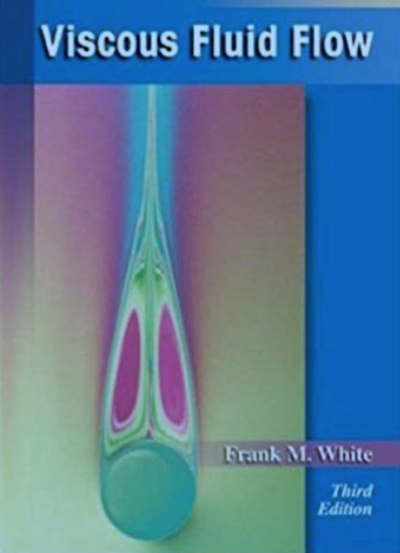#### ADVANCED FLUID MECHANICS / VISCOUS FLOW (MKMM 1313)

IMPORTANT ANNOUNCEMENT !!

All recorded video during the class will be uploaded in YouTube channel. Please subscribe my channel to get notification. You may also go to my channel and watch previous video notes in playlist.

Choose "1313" in my playlist to watch video notes for Advanced Fluid Mechanics (also known as Viscous Fluid Flow)

• ### COURSE INFORMATION

Open or Close
This course is intended for graduate students wishing to have deep understanding in viscous flow and boundary layer. In this course, it starts with basic properties of fluid and derivation of basic equations for simple viscous flow problems. Student also will be introduced the boundary condition for viscous flow problems. Then, the derivation of basic equation of viscous flow regarded to conservation of mass, conservation of momentum and energy equation will be introduced. After that, a set of equations based from the conservation of momentum were used to solve Couette flow and Poiseuille flow. Finally, applying the momentum equation to solve boundary layer related engineering problems and incompressible turbulent mean flow problems.

1. Derive basic equation of viscous flow regarded to conservation of mass, momentum and energy equations.
2. Apply governing equations for viscous flow to solve Couette flow and Poiseuille flow.
3. Apply momentum equation to solve boundary layer related engineering problems.
4. Derivation and solving problem for incompressible turbulent mean flow.

• ### SYLLABUS

Open or Close
BASIC EQUATION OF FLOW:
Classification of the fundamental equations.
Conservation of mass: The continuity equation.
Conservation of momentum: The Navier-Stokes equation.
The energy equation: First law of thermodynamics.

VISCOUS FLOW:
Introduction and classification of solution.
Couette flow due to moving surfaces.
Poiseuille flow through ducts.

LAMINAR BOUNDARY LAYER:
Laminar boundary layer equations
Similarity solutions for steady two-dimensional flow.
Three-dimensional laminar boundary layers.
Flow in the inlet of duct.

TURBULENT FLOW:
Physical and mathematical description of turbulence.
The two-dimensional turbulent boundary layer equations.
The turbulent boundary layer on a flat plate.

• ### ASSESSMENT

Open or Close
Test 1 - 20%
Test 2 - 20%

Assignment - 20% (You will have 4 assignments)

Final Exam - 40%

(Marks percentage may be changed due to Fluid Panel's decision)
• ### REFERENCES

Open or CloseOfficial Text book

Viscous Fluid Flow.
Frank M. White.
Third Edition, Mc Graw-Hill.
Other References
1. Munson, Young, Okiishi, Huebsch. 2010. Fundamental of Fluid Mechanics, 6th Ed. John Wiley and Sons.
2. Fox, McDonald and Pritchard, 2004, Introduction to Fluid Mechanics, 6th Edition, John Wiley and Sons.
3. Douglas, Gasiorek, Swaffield, Fluid Mechanics, 4th Edition, Prentice Hall.
4. White. 2016. Fluid Mechanics. 8th Edition. McGraw-Hill International Edition.
5. Massey, Mechanics of Fluids, Chapman & Hall, London.